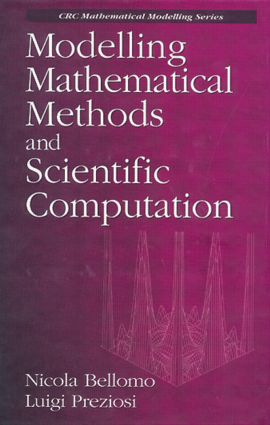# Modelling Mathematical Methods and Scientific Computation

## 1st Edition

CRC Press

512 pages

##### Purchasing Options:\$ = USD
Hardback: 9780849383311
pub: 1994-12-22
\$230.00
x

FREE Standard Shipping!

### Description

Addressed to engineers, scientists, and applied mathematicians, this book explores the fundamental aspects of mathematical modelling in applied sciences and related mathematical and computational methods. After providing the general framework needed for mathematical modelling-definitions, classifications, general modelling procedures, and validation methods-the authors deal with the analysis of discrete models. This includes modelling methods and related mathematical methods. The analysis of models is defined in terms of ordinary differential equations. The analysis of continuous models, particularly models defined in terms of partial differential equations, follows. The authors then examine inverse type problems and stochastic modelling. Three appendices provide a concise guide to functional analysis, approximation theory, and probability, and a diskette included with the book includes ten scientific programs to introduce the reader to scientific computation at a practical level.

### Reviews

"The style of presentation is simply excellent. The authors carefully separate definitions, examples, theory, and remarks. This organization makes this text highly readable, suitable for use as both a text and a reference."

- F. Jafari, Appl. Mech. Rev., 49(5), May 1996

Preface

Mathematical Modelling

Introduction

Definition of Mathematical Modelling

Classification of Mathematical Modelling

Modelling Methods

Validation of Mathematical Models

Mathematical Modelling as a Science

Discrete Models

Plan of Chapter 2

Mathematical Formulation of Problems

On Existence, Uniqueness and Continuity

Linear Systems

Stability and Linearization

From Bifurcation to Chaos

Numerical Methods for Initial Value Problems

Scientific Programs

Continuous Models

Introduction

Mathematical Modelling

Equilibrium Equation for the Vibration of an Elastic String

Mathematical Models of Continuum Mechanics

Mathematical Models of Electromagnetism

Direct Simulation Models in Biology

Classification and Characteristics

Mathematical Formulation of Problems

Finite Difference Methods

The Collocation Method

Decomposition of Domains

Applications and Scientific Programs

Inverse and Stochastic Problems

Inverse Problems and Stochastic Models

Classification of Inverse Problems

Solution by Decomposition of Domains

Solution by Minimization Techniques

Mathematical Modelling and Stochasticity

Classification of Discrete Stochastic Models

Classification of Continuous Stochastic Models

Modelling and Solution of Problems

Stochastic Aspects and Inverse Problems

Kinetic Models

Application

Discussion and Developments

Scientific Programs

Appendix 1. Functional Spaces and Fixed Point Theorems

Appendix 2. Interpolation and Approximation

Appendix 3. Random Variables

References

Subjects Index

### Subject Categories

##### BISAC Subject Codes/Headings:
MAT000000
MATHEMATICS / General
MAT021000
MATHEMATICS / Number Systems